Function Repository Resource:

# ErikssonPolarAngle

Multidimensional polar angle of a corner

Contributed by: Michael Reed
 ResourceFunction["ErikssonPolarAngle"][o,{p1,…,pd}] gives the polar angle at the point o and spanned by the vectors p1,…,pd.

## Details

ResourceFunction["ErikssonPolarAngle"] generalizes the law of sines for d-dimensional spherical simplices.

## Examples

### Basic Examples (2)

The polar angle at the origin for vectors in two dimensions:

 In:=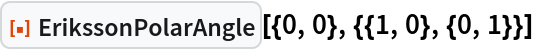Out=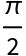The polar angle at the origin for vectors in three dimensions:

 In:=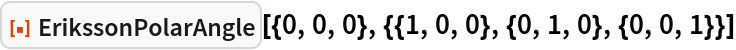Out=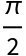### Scope (1)

ErikssonPolarAngle works in any number of dimensions:

 In:=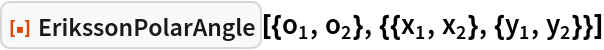Out=In:=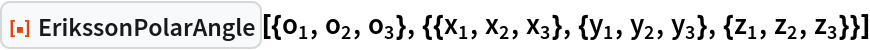Out=In:=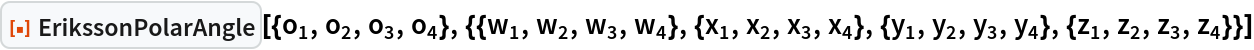Out=### Properties and Relations (2)

In two dimensions, ErikssonPolarAngle is similar to the results of SolidAngle and PlanarAngle:

 In:=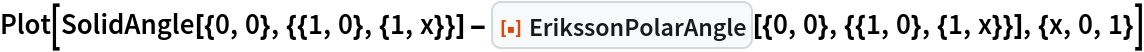Out=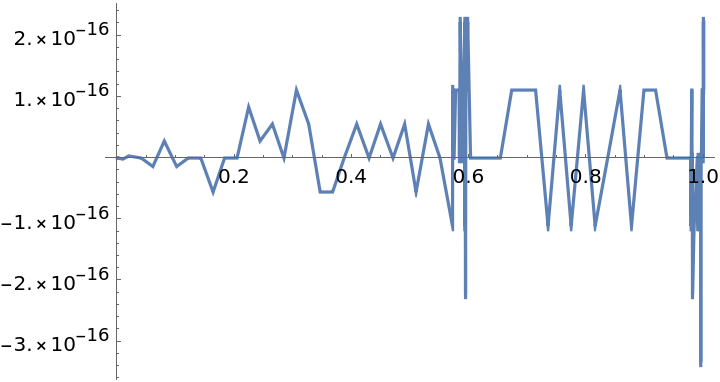In three dimensions, the difference between ErikssonPolarAngle and SolidAngle is more apparent:

 In:=Out=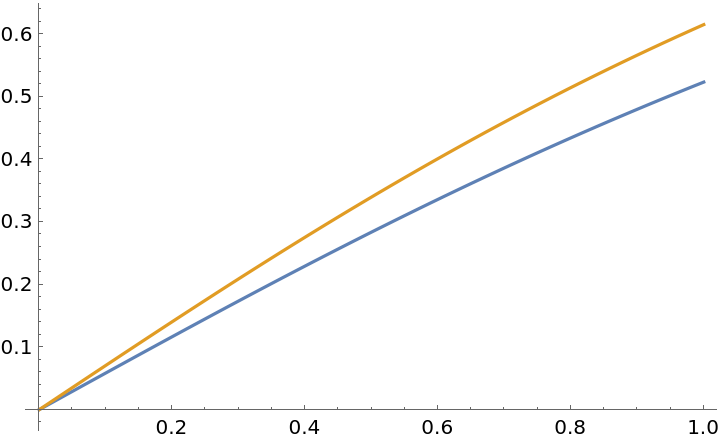Michael Reed

## Version History

• 1.0.0 – 20 July 2022